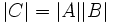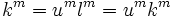# Euler-phi function is multiplicative if coprime

## Statement

### Verbal statement

The Euler-phi function is multiplicative on coprime natural numbers.

### Statement with symbols

For any natural numbers$m,n$ that are coprime to each other,$\varphi(mn)=\varphi(m)\varphi(n)$.

## Proof

Given:$m,n$
coprime positive integers


To prove:$\varphi(mn)=\varphi(m)\varphi(n)$ Proof: Consider a finite cyclic group G of order$mn$. Denote by$A,B,C$ the set of all elements in G that have orders$m,n,mn$ respectively. We will show that$|C|=|A||B|$.
Define a map$\phi : A \times B \rightarrow C$ by$\phi((g,h))=gh$. Since the order of$g$ and$h$ are coprime, and$G$ is abelian, their product$gh$ must have order$mn$, so this map is well-defined. If$\phi((g_1,h_1))=\phi((g_2,h_2))$ then$g_1h_1=g_2h_2$, or equivalently,$g_2^{-1}g_1=h_2h_1^{-1}$. They are elements of subgroups of coprime orders that must intersect trivially. So,$g_1=g_2$ and$h_1=h_2$, the map is injective. Thus,$|A \times B| \le |C|$.
Define another map$\psi : C \rightarrow A \times B$ by$\psi(k)=(k^{n},k^{m})$. If$\psi(k)=\psi(l)$ then$k^{n}=l^{n}$ and$k ^ m = l ^ m$. Suppose$k=ul$. Then$k ^ m = u ^ m l ^ m = u ^ m k ^ m$. Therefore$u ^ m = e$. Similarly,$u ^ n = e$. So,$m$ and$n$ must have a common divisor which is the order of$u$. Thus,$u=e$. Hence our map is again injective, so$|C| \le |A \times B|$.
Therefore,$|C|=|A \times B|=|A||B|$, as desired.